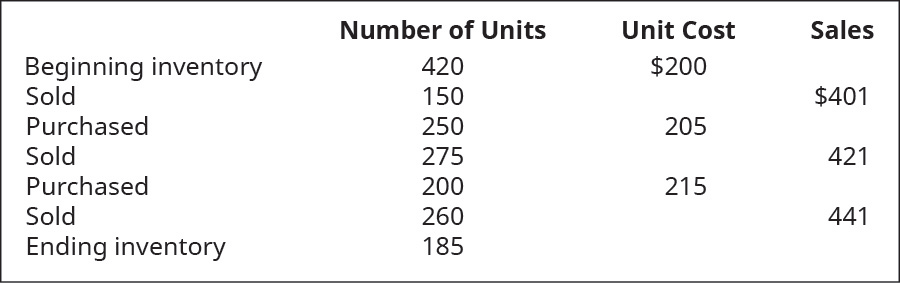# Calculate a) cost of goods sold, b) ending inventory, and c) gross margin for B76 Company, considering the following transactions under three different cost allocation methods and using perpetual inventory updating. Provide calculations for last-in, first-out (LIFO).FindFindarrow_forward

### Principles of Accounting Volume 1

19th Edition
OpenStax
Publisher: OpenStax College
ISBN: 9781947172685

#### Solutions

Chapter
SectionFindFindarrow_forward

### Principles of Accounting Volume 1

19th Edition
OpenStax
Publisher: OpenStax College
ISBN: 9781947172685
Chapter 10, Problem 10PB
Textbook Problem
1 views

## Calculate a) cost of goods sold, b) ending inventory, and c) gross margin for B76 Company, considering the following transactions under three different cost allocation methods and using perpetual inventory updating. Provide calculations for last-in, first-out (LIFO).To determine

Introduction:

Last In First Out is a method of evaluation of cost of goods sold. In this method, it is assumed that units purchased at last is sold first. Costs in relation with units sold are taken from cost of last lot and in case, the number of sold units is higher than the latest lot purchased, then the cost of last second lot is used.

To compute:

The COGS, ending inventory and gross margin under three different cost allocation methods using perpetual inventory system. Also, show calculations for LIFO.

### Explanation of Solution

Table showing COGS, ending inventory, and gross margin under three different cost allocation methods using perpetual inventory system:

 Particulars LIFO ($) FIFO ($) Weighted Average ($) COGS2 141,250 138,475 139,757 Ending Inventory1 37,000 39,775 38,492 Gross Margin3 149,335 152,110 150,828 Table (1) Working Notes: 1. Computation of Closing Inventory:  Particulars Amount ($) Opening Inventory ((420−150−25−60)×$200) 37,000 Lot-1 Purchases ((250−250)×$205) 0 Lot-2 Purchases ((200−200)×\$215) 0 Total 37,000

Table (2)

2

### Still sussing out bartleby?

Check out a sample textbook solution.

See a sample solution

#### The Solution to Your Study Problems

Bartleby provides explanations to thousands of textbook problems written by our experts, many with advanced degrees!

Get Started